Test: Basic Concepts - 1

# Test: Basic Concepts - 1

Test Description

## 10 Questions MCQ Test Network Theory (Electric Circuits) | Test: Basic Concepts - 1

Test: Basic Concepts - 1 for Electrical Engineering (EE) 2022 is part of Network Theory (Electric Circuits) preparation. The Test: Basic Concepts - 1 questions and answers have been prepared according to the Electrical Engineering (EE) exam syllabus.The Test: Basic Concepts - 1 MCQs are made for Electrical Engineering (EE) 2022 Exam. Find important definitions, questions, notes, meanings, examples, exercises, MCQs and online tests for Test: Basic Concepts - 1 below.
Solutions of Test: Basic Concepts - 1 questions in English are available as part of our Network Theory (Electric Circuits) for Electrical Engineering (EE) & Test: Basic Concepts - 1 solutions in Hindi for Network Theory (Electric Circuits) course. Download more important topics, notes, lectures and mock test series for Electrical Engineering (EE) Exam by signing up for free. Attempt Test: Basic Concepts - 1 | 10 questions in 30 minutes | Mock test for Electrical Engineering (EE) preparation | Free important questions MCQ to study Network Theory (Electric Circuits) for Electrical Engineering (EE) Exam | Download free PDF with solutions
 1 Crore+ students have signed up on EduRev. Have you?
Test: Basic Concepts - 1 - Question 1

### A solid copper sphere, 10 cm in diameter is deprived of 1020 electrons by a charging scheme. The charge on the sphere is

Detailed Solution for Test: Basic Concepts - 1 - Question 1

n = 1020 ,  Q =  ne = 1020 e (e = 1.6 x 10-19 )
= 16.02 C
The charge on the sphere is 16.02 coulomb. It will be positive.

Test: Basic Concepts - 1 - Question 2

### A lightning bolt carrying 15,000 A lasts for 100 μs. If the lightning strikes an airplane flying at 2 km, the charge deposited on the plane is

Detailed Solution for Test: Basic Concepts - 1 - Question 2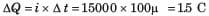Test: Basic Concepts - 1 - Question 3

### If 120 C of charge passes through an electric conductor in 60 sec, the current in the conductor is

Detailed Solution for Test: Basic Concepts - 1 - Question 3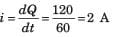Test: Basic Concepts - 1 - Question 4

The energy required to move 120 coulomb through 3V is

Detailed Solution for Test: Basic Concepts - 1 - Question 4

W = Qv = 360J

Test: Basic Concepts - 1 - Question 5

i = ?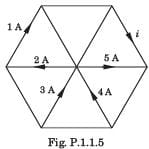Detailed Solution for Test: Basic Concepts - 1 - Question 5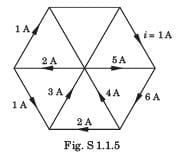Test: Basic Concepts - 1 - Question 6

In the circuit of fig P1.1.6 a charge of 600 C is delivered to the 100 V source in a 1 minute. The value of v1 must be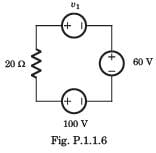Detailed Solution for Test: Basic Concepts - 1 - Question 6

In order for 600 C charge to be delivered to the 100 V source, the current must be anticlockwise.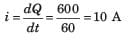Applying KVL we get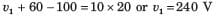Test: Basic Concepts - 1 - Question 7

In the circuit of the fig P1.1.7, the value of the voltage source E is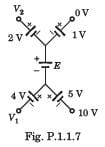Detailed Solution for Test: Basic Concepts - 1 - Question 7

Going from 10 V to 0 V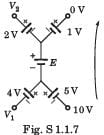10 + 5 + E + 1 + 0 = 0 or E =-16 V

Test: Basic Concepts - 1 - Question 8

Consider the circuit graph shown in fig. P1.1.8. Each branch of circuit graph represent a circuit element. The value of voltage v1 is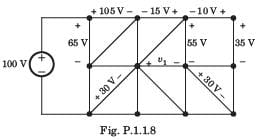Detailed Solution for Test: Basic Concepts - 1 - Question 8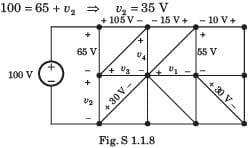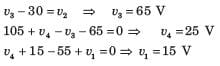Test: Basic Concepts - 1 - Question 9

For the circuit shown in fig P.1.1.9 the value of voltage vo is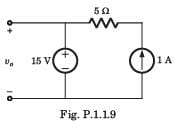Detailed Solution for Test: Basic Concepts - 1 - Question 9

Voltage is constant because of 15 V source.

Test: Basic Concepts - 1 - Question 10

R1 = ?​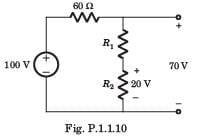Detailed Solution for Test: Basic Concepts - 1 - Question 10

Voltage across 60 Ω resistor = 30 V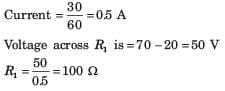## Network Theory (Electric Circuits)

23 videos|21 docs|27 tests
 Use Code STAYHOME200 and get INR 200 additional OFF Use Coupon Code
Information about Test: Basic Concepts - 1 Page
In this test you can find the Exam questions for Test: Basic Concepts - 1 solved & explained in the simplest way possible. Besides giving Questions and answers for Test: Basic Concepts - 1 , EduRev gives you an ample number of Online tests for practice

## Network Theory (Electric Circuits)

23 videos|21 docs|27 tests